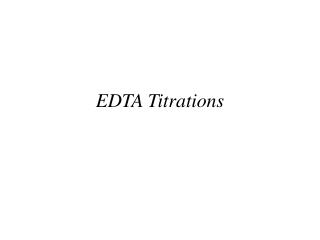Download PresentationEDTA Titrations

# EDTA Titrations

Download Presentation## EDTA Titrations

- - - - - - - - - - - - - - - - - - - - - - - - - - - E N D - - - - - - - - - - - - - - - - - - - - - - - - - - -
##### Presentation Transcript

1. EDTA Titrations

2. Chelation in Biochemistry Chelating ligands can form complex ions with metals through multiple ligands. This is important in many areas, especially biochemistry.

3. Metal-Chelate Complexes • Metals are Lewis acids that accept electron pairs from donating ligands that act as Lewis bases • CN- is a common monodentate ligand, binding to a metal ion through one atom (C) • Metals can bind to multiple ligands (usually 6) • A ligand that can attach to a metal by more than one atom is multidentate or a chelating ligand • Chelating agents can be used for titration of metals to form complex ions (complexometric titration)

4. Chelating Agents in Analytical Chemistry

5. Ethylenediamenetetraacetic acid (EDTA) EDTA forms 1:1 complexes with metal ions by with 6 ligands: 4 O & 2N. EDTA is the most used chelating agent in analytical chemistry, e.g. water hardness.

6. Acid/Base Properties of EDTA • EDTA is a hexaprotic system (H6Y2+) with 4 carboxylic acids and 2 ammoniums: • We usually express the equilibrium for the formation of complex ion in terms of the Y4- form (all six protons dissociated). You should not take this to mean that only the Y4- form reacts

7. Fraction of EDTA in Y4- Form • Similar to acids and bases, we can define fractional compositions, α, defined as the fraction of “free” EDTA in a particular form. • “Free” means uncomplexed EDTA • So, for Y4-:

8. EDTA Complexes • The equilibrium constant for a reaction of metal with EDTA is called the formation constant, Kf, or the stability constant: • Again, Kf could have been defined for any form of EDTA, it should not be understood that only the Y4- reacts to form complex ion.

9. pH Dependence of αY4-

10. Formation Constants for M-EDTA Complexes

11. Some Metals Form 7 or 8 Coordinate Complexes The rings formed in the M-EDTA complex can become strained. If the oxygen atoms pull back toward the nitrogen atoms, the strain is relieved. This opens up the metal to other ligands. Water molecules frequently occupy these sites.

12. Conditional Formation Constant • We saw from the fraction plot that most of the EDTA is not in the form of Y4- below a pH ~10. • We can derive a more useful equilibrium equation by rearranging the fraction relationship: • If we fix the pH of the titration with a buffer, then αY4- is a constant that can be combined with Kf

13. Example • Calculate the concentration of free Ca2+ in a solution of 0.10 M CaY2- at pH 10 and pH 6. Kf for CaY2- is 4.9x1010 (Table 13-2) • At low pH, the metal-complex is less stable

14. Calcium/EDTA Titration Curve For calcium, the end point becomes hard to detect below ~pH=8. The formation constant is too small below this point. This can be used to separate metals. At pH=4, Ca does not perform significant complexaion with EDTA. However, Fe can still form the complex, so it can be titrated without interference from Ca.

15. Generic Titration Curve Like a strong acid/strong base titration, there are three points on the titration curve of a metal with EDTA: before, at, and after the equivalence point. We’ll consider a titration where we have 50.0 mL of 0.040 M Ca2+ (buffered at pH=10) with 0.080 M EDTA. Ve=25.0 mL

16. Before the Equivalence Point • What’s pCa2+ when we have added 5.0 mL of EDTA? Dilution Factor Fraction Remaining Initial Concentration

17. At the Equivalence Point • What’s pCa2+ when we have added 25.0 mL of EDTA? • At the equivalence point almost all the metal is in the form CaY2- • Free Calcium is small and can be found w/ algebra Dilution Factor Initial Concentration

18. After the Equivalence Point • What’s pCa2+ when we have added 26.0 mL of EDTA? • We have 1.0 mL excess EDTA Initial Concentration Dilution Factor Initial Concentration Dilution Factor

19. Auxiliary Complexing Agents • In aqueous solution, metal-hydroxide complexes or precipitates can form, especially at alkaline pH • We often have to use an auxiliary complexing agent • This is a ligand that binds strongly enough to the metal to prevent hydroxide precipitation, but weak enough to be displaced by EDTA • Ammonia is a common auxiliary complex for transition metals like zinc

20. Metal Ion Indicators • To detect the end point of EDTA titrations, we usually use a metal ion indicator or an ion-selective electrode (Ch. 15) • Metal ion indicators change color when the metal ion is bound to EDTA: • Eriochrome black T is an organic ion • The indicator must bind less strongly than EDTA

21. Metal Ion Indicator Compounds

22. EDTA Titration Techniques • Direct titration: analyte is titrated with standard EDTA with solution buffered at a pH where Kf’ is large • Back titration: known excess of EDTA is added to analyte. Excess EDTA is titrated with 2nd metal ion.

23. EDTA Titration Techniques (2) • Displacement titration: For metals without a good indicator ion, the analyte can be treated with excess Mg(EDTA)2-. The analyte displaces Mg, and than Mg can be titrated with standard EDTA • Indirect titration: Anions can be analyzed by precipitation with excess metal ion and then titration of the metal in the dissolved precipitate with EDTA.

24. Example Titration • 25.0 mL of an unknown Ni2+ solution was treated with 25.00 mL of 0.05283 M Na2EDTA. The pH of the solution was buffered to 5.5 and than back-titrated with 17.61 mL of 0.02299 M Zn2+. What was the unknown Ni2+ M?